Select Page

# Conversion From Moore to Mealy Machine

Each Moore Machine is easy converted into its corresponding Mealy Machine. The equivalence of the Moore and Mealy machine means that both the machines produce the same output for same input.

As we know that, in Moore machine, the output is attached with every state symbol, and in the mealy machine, the output is attach with input symbol.

## Method To Convert Moore to Mealy Machine

Find the output symbol of each transition from each state. And simply place the output symbol along with input over arrow.

Above procedure start from initial state and repeat till the end. Simple Moore to Mealy Machine is examples are given below.

## Example 01

Consider the following Moore MachineLet’s convert above Moore Machine into corresponding Mealy Machine.

At q0 for input “0”, transition also goes the state q0 which gives output “a”. So for Mealy Machine, Just label output (a) with input (1) over arrow as given below.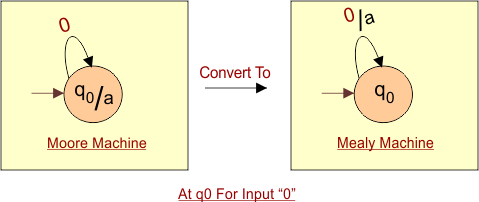At q0 for input “1”, transition also goes the state q0 which gives output “a”. So for Mealy Machine, Just label output (a) with input (1) over arrow as given below.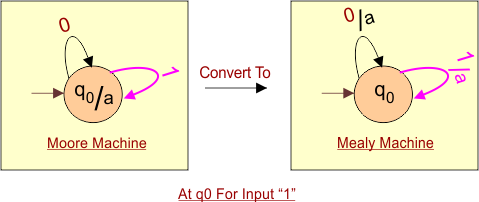Hence in above diagram, the given Moore Machine is converted into corresponding Mealy Machine.

## Example 2

Consider the following Moore Machine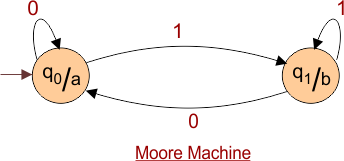### Solution

At q0 for input “0”, transition also goes the state q0 which gives output “a”. So for Mealy Machine, Just label output with input over arrow as given below.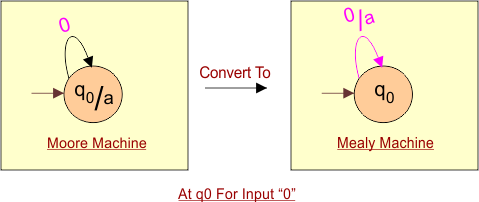At q0 for input “1”, transition also goes the state q1 which gives output “b”. So for Mealy Machine, Just label output (b) with input (1) over arrow as given below.Now Move to q1 to discuss both inputs “0” and “1”.

At q1 for input “0”, transition also goes to the state q1 which gives output “b”. So for Mealy Machine, Just label output (b) with input (1) over arrow as given below.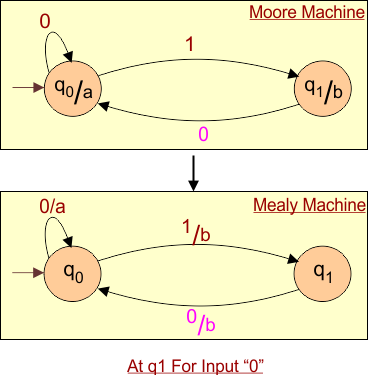At q1 for input “1”, transition goes to the state q0 which gives output “a”. So for Mealy Machine, Just label output (b) with input (0) over arrow as given below.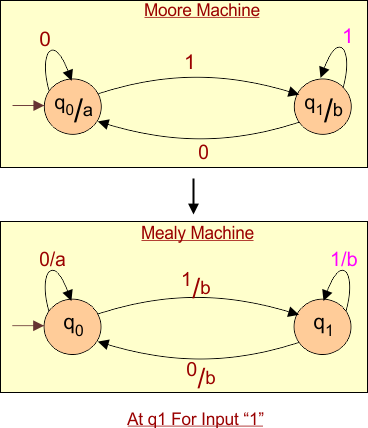Hence in above diagram, the given Moore Machine is converted into corresponding Mealy Machine.

## Example 03

Convert the following Moore machine into its equivalent Mealy machine.

### Solution

After applying conversion rules which we see in above examples, result in Mealy Machine is given below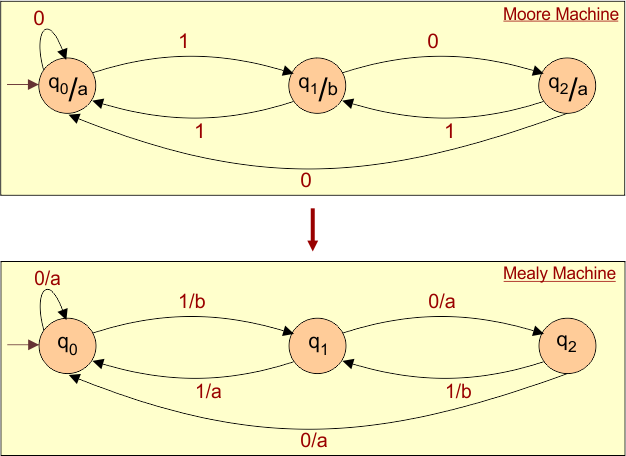# Important

Number of states

After conversion from Moore to Mealy Machine, The states of Mealy Machine are always less than or equal to Moore Machine.

Transition Table

Transition table can also be obtained from corresponding Moore or Mealy diagrams. As we discus early in the Moore and Mealy Machine lectures.

Help Other’s By Sharing…